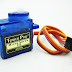I have already covered a tutorial about Pulse Width Modulation in STM32 HERE and in this tutorial i am going to cover one of its applications. So guys today I am going to show you how to control servo motor with STM32 by using PWM.

Servo motors use feedback to determine the position of the shaft, you can control that position very precisely. As a result, servo motors are used to control the position of objects, rotate objects, move legs, arms or hands of robots, move sensors etc. with high precision.
Most servo motors have the following three connections:
• Black/Brown ground wire.
• Red power wire (around 5V).
• Yellow or White PWM wire.

## HOW IT WORKS

Servos can go from 0 to 180 degrees depending on the width of the pulse. Basically you need to keep the pulse (+5v) high  for particular amount of time. Few are listed below:

•  1 milliseconds and corresponds to 0 degrees
• 1.5 milliseconds and corresponds to 90 degrees
• 2 milliseconds and corresponds to 180 degrees

The period between two pulses must be 20ms or we can say the frequency of the pulse must be 50 Hz.

## How to program

This is very important part because it involves messing up with the timers. I know it’s very hard to understand what is what when it comes to timers. So I am going to keep it simple here so that you guys can understand what’s going on.

I am going to use TIM2 of my STM32F446RE. TIM2 is connected to APB1 bus which gives me timer clock max upto 90 MHz. But the prescalar register is only 16 bit so max value I can write there is 65535. Keeping this in mind, I am going to set the clock such that my TIM2 gets only 45 MHz. my setup is below:

Okay now as I mentioned above that the frequency must be 50 Hz, it is time to divide the clock using prescalar and the ARR registers. Let’s do some calculations than–>  (45 MHz/50 Hz) = 900 KHz. So now to get a  50 Hz of frequency, I have to divide this 900 KHz between Prescalar and ARR. So I am going to write 900 to prescalar Register and 1000 to ARR. The reason behind this is this 1000 will also act as 1000% pulse width and if I want to change the pulse width to any other value, all I have to do is write X% to CCR1 register. This simplifies the things a lot.
For example, If I want to give a pulse width of 1 ms i.e. (1*1000/20) = 50%, I will write 50 instead of X in CCR1 register. For 2 ms, It will be 100%, and for 1.5 ms, It will be 75% and so on..
After testing it from 1 ms to 2ms, I found that the servo was not rotating the full 180 degrees as it should So I decided to take it a little further and tested it from 0.5 ms to 2.5 ms and It performed well. So the code below is for 0.5 to 2.5 ms, However I commented out the section for 1 to 2 ms.

## Some Insight Into the CODE

start the timer in PWM mode
``HAL_TIM_PWM_Start(&htim2, TIM_CHANNEL_1);``

Write Values

``````while (1)
{
htim2.Instance->CCR1 = 25;  // duty cycle is .5 ms
HAL_Delay(2000);

htim2.Instance->CCR1 = 75;  // duty cycle is 1.5 ms
HAL_Delay(2000);

htim2.Instance->CCR1 = 125;  // duty cycle is 2.5 ms
HAL_Delay(2000);
}
``````

Servo motor with STM32Reviewed by arun singh on April 01, 2018 Rating: 5